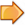Backgammon Theory# Is Brute Force Backgammon Possible? David Levner, 1976

Computer Science Department
Carnegie-Mellon University, Pittsburgh, PA 15213

 Originally published in SIGART Newsletter, no. 58 (June 1976), 20.

In view of the recent interest in backgammon, two mathematical questions can be asked: how many backgammon positions are there, and how hard would it be to solve by brute force?

The first question shall be answered here, but the second is very difficult. Von Neumann and Morgenstern1 solved all finite zero-sum games of perfect information of bounded length; but backgammon can continue arbitrarily long since it is possible to repeat a position arbitrarily often; worse, backgammon is not a finite game because the players can double the stakes any number of times. No known algorithm computes the optimal strategy, nor has anyone proven that such a strategy exists.

The number of backgammon positions is much easier to compute. The number of times the players have doubled cannot be considered for then there would be infinitely many positions. Only the three states of possession of the doubling cube (it can belong to player one, player two, or both players) are counted.

A backgammon board consists of three areas: white’s offboard (which is also black’s bar), black’s offboard (which is also white’s bar, and the twenty-four points of the backgammon board. There are fifteen white men and fifteen black. Men of opposite color can coexist in all areas, but they cannot coexist on any of the 24 points. A backgammon position is determined by seven factors: the player on the move, the state of possession of the doubling cube, the numbers of white men on the bar, or borne off, the numbers of black men on on the bar, or borne off, and the occupation of the 24 points by the remaining men.

It is useful to define B(m, n), the number of ways to arrange m men of a single color on n points (or n areas). To calculate B(m, n), m+1 cases are distinguished: case i (0 ≤ im) corresponds to placing i men on the first point, leaving mi men to be arranged on n−1 points, which can be done in B(mi, n−1) ways. Summing the m+1 cases yields equation (1):

 (1) B(m, n)  = mΣi = 0 B(m−i, n−1)

Note that every position is counted once and only once. Table 1 lists the values of B(m, n) for m = 0…5 and n = 1…6.

Table 1

 m 0 1 2 3 4 5 n 1 1 1 1 1 1 1 2 1 2 3 4 5 6 3 1 3 6 10 15 21 4 1 4 10 20 35 56 5 1 5 15 35 70 126 6 1 6 21 56 126 252

There are B(15, 3) ways to allot the white men among the three areas, and just as many ways for the black, for a total of B(13, 3)2 = 1362 = 18496 possibilities. Each possibility leaves a certain number of men, say w white men and b black men, to be distributed over the 24 points.

To compute the number of occupations of w white men and b black men on 24 points, w cases are distinguished. Case i (1 ≤ iw) corresponds to placing the w white men on exactly i points. At least one white man must occupy each of the i points, and the reamining wi men can be arranged on the i points in B(wi, i) ways. There are

 ( 24i )

sets of i points. Once the white men are in place, B(b, 24−i) arrangements of the b black men on 24−i points are possible. Hence case i contains a total of

 ( 24i ) B(w−i, i) B(b, 24−i)

occupations of the white and black men. The full number is obtained from the sum of the w cases:

 (2) oc(w, b)  = wΣi = 1 ( 24i ) B(w−i) B(b, 24−i)

Every occupation has been counted exactly once.

The number of backgammon positions (BKG) is calculated by equation (3).

 (3)    BKG  = Σfor all p, d, woff, wbar, boff, bbar oc(15−woff−wbar, 15−boff−bbar)

where p is a player, d is a state pof possession of the doubling cube, woff is the number of white men borne off, wbar the number of white men on the bar, and boff and bbar are defined similarly. Note that there are two players and three possible states of the doubling cube.  BKG was calculated from these three equations and found to be 1.1 × 1020.

1. Morgenstern, O. and Von Neumann, J. The Theory of Games and Economic Behavior, Princeton University Press, Third edition, Princeton NJ, 1953.Other articles by David LevnerOther articles on backgammon theory Return to:  Backgammon Galore